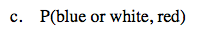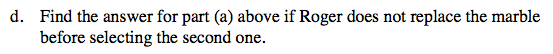### Home > CC2 > Chapter 8 > Lesson 8.1.1 > Problem8-18

8-18.
1. Roger has a bag of marbles. There are 6 red, 4 blue, 3 white, and 7 green marbles in the bag. If he draws one marble, replaces it, and then draws another, find the following probabilities. Write your answer as a fraction and a percent. Homework Help ✎

1. P(red, red)

2. P(not white, green)

3. P(blue or white, red)

4. Find the answer for part (a) above if Roger does not replace the marble before selecting the second one.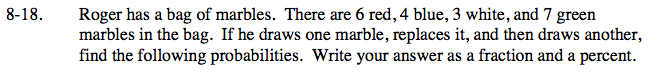Find the number of specified marbles out of the entire set of marbles and write it as a fraction probability.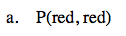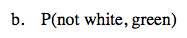$\frac{119}{400}=29.75\%$